# I can define forward reaction, reverse reaction, reversible reaction, and closed system

 Page 14/19 Date 23.04.2018 Size 1.3 Mb. #45980
 _____26. I can define forward reaction, reverse reaction, reversible reaction, and closed system Definitions: forward reaction – the chemical reaction read from left to right reverse reaction – the chemical reaction read from right to left reversible reaction – a chemical reaction that can proceed from both left to right and right to left closed system – a system in which reactants and products are trapped and may not enter or leave _____27. I can state the three types of equilibrium. The three types of equilibrium are: ___Phase______ equilibrium ____Chemical/reaction_______ equilibrium and ____Solution___________________ equilibrium

_____28. I can state two conditions that apply to all systems at equilibrium.

In a system at equilibrium the ____rate__________ of the forward and reverse reaction must be ____equal____________ and the ______concentrations_____________ of the reactants and products must be ______constant______.

_____29. Given a list of reactions, I can identify reactions that show equilibrium (chemical, phase, or solution).

Which balanced equation represents phase equilibrium?

A) H2(g) + I2(s) <-----> 2HI(g)

H2O
B) I2(s) <-----> I2(g)

C) KCl(s) <-----> KCl(aq)

D) 2KCl(s) + 3O2(g) -----> 2KClO3

Which balanced equation represents solution equilibrium?

A) H2(g) + I2(s) <-----> 2HI(g)

H2O
B) I2(s) <-----> I2(g)

C) KCl(s) <-----> KCl(aq)

D) 2KCl(s) + 3O2(g) -----> 2KClO3

Which balanced equation represents chemical equilibrium?

A) H2(g) + I2(s) <-----> 2HI(g)

H2O
B) I2(s) <-----> I2(g)

C) KCl(s) <-----> KCl(aq)

D) 2KCl(s) + 3O2(g) -----> 2KClO3

_____30. In terms of saturation, I can describe a solution that is at equilibrium.

In terms of saturation, a solution that is at equilibrium must be
____saturated_________.

_____31. I can state LeChatelier’s Principle.

LeChatelier’s Principle states when subjected to a stress, systems at equilibrium will shift to relieve the stress

_____32. Given a balanced equation at equilibrium, I can predict the direction of shift in the equilibrium when the temperature, concentration, or pressure is changed or if a catalyst is added.

Given the reaction at equilibrium:
2SO2(g) + O2(g) <-----> 2SO3(g) + 392kJ
Predict the direction of shift in the equilibrium (right, left, no shift) when the following changes are made to the system.
 Change Direction of Shift Increase concentration of SO2 right Increase concentration of SO3 left Increase temperature left Increase pressure right Add a catalyst no shift

Unit 13: Organic Chemistry

If you can do all the things listed below, you are ready for the Unit 13 test.

Place a checkmark next to each item that you can do! If a sample problem is given, complete it as evidence.
 _____1. I can still do everything from Unit 1. _____2. I can still do everything from Unit 2. _____3. I can still do everything from Unit 3. _____4. I can still do everything from Unit 4. _____5. I can still do everything from Unit 5. _____6. I can still do everything from Unit 6. _____7. I can still do everything from Unit 7. _____8. I can still do everything from Unit 8. _____9. I can still do everything from Unit 10. _____10. I can still do everything from Unit 11. _____11. I can still do everything from Unit 12. _____12. I can define organic compound, saturated hydrocarbon, unsaturated hydrocarbon, and isomer. Definitions: organic compound – all carbonate containing compounds except CO, CO2, and carbonates saturated hydrocarbon – a compound containing only C and H in which each carbon atom has 4 single bonds unsaturated hydrocarbon - a compound containing only C and H in which there is at least one double bond (possibly triple) between C atoms isomer –two organic compounds that have the same molecular formula, but different structural formula and therefore different physical and chemical properties

 _____13. I can expand a condensed structural formula to show the structural formula of an organic compound. Draw the complete structural formula for CH3CH2CH2CH2CH3. (Sorry. This will take WAAY too long on the computer.) Draw the complete structural formula for CH3CHCHCH3. (This one too.) _____14. I can state the name and symbol of the element that is capable of forming rings, chains, and networks. The element that is capable of forming rings, chains, and networks is ____carbon______. Its symbol is_______C_______. _____15. I can explain the meaning of and apply HONC1234. HONC1234 tells me that Hydrogen forms 1 bond. Oxygen forms 2 bonds. Nitrogen forms 3 bonds. Carbon forms 4 bonds.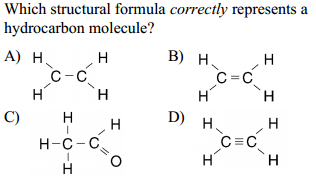_____16. Given the formula, I can determine if a compound is a hydrocarbon or not.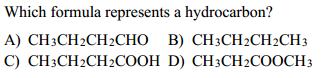_____17. Given the name, I can use Reference Table P to determine how many carbons atoms are in a compound. Determine how many carbon atoms are in each of the following compounds: decane_______10________________ ethene_________2_________________ 3-nonene______9_______________ 1-pentyne________5_________________ _____18. Given the name, I can use Reference Table Q to determine to which class of hydrocarbons a compound belongs. Determine the homologous series of hydrocarbons to which each of the following belongs: decane____alkane_______________ 2-decene______alkene____________ 3-nonene___alkene_____________ 1-pentyne_______alkyne____________ _____19. Given the name, I can determine if the hydrocarbon is saturated or unsaturated. Determine if each of the following is a saturated or unsaturated hydrocarbon. decane_______sat_________ ethene_________unsat________________ 3-nonene_____unsat____________ 1-pentyne_____unsat_______________ _____20. Given the formula, I can determine to which homologous series a hydrocarbon belongs. Determine the homologous series of hydrocarbons to which each of the following belongs: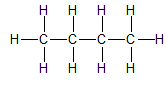belongs to the ____alkane____________ series.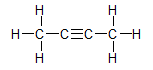belongs to the ________alkyne__________ series.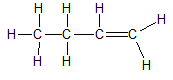belongs to the _____alkene____________ series.